Get instant live expert help with Excel or Google Sheets“My Excelchat expert helped me in less than 20 minutes, saving me what would have been 5 hours of work!”

#### Post your problem and you'll get expert help in seconds

Your message must be at least 40 characters
Our professional experts are available now. Your privacy is guaranteed.

# How to use the Excel ODD function

We use the Excel ODD function, which is a built-in function to round up a given number and return the next odd integer. With this function, we round up numbers such that positive numbers become larger and negative numbers become smaller. This article will walk through the process.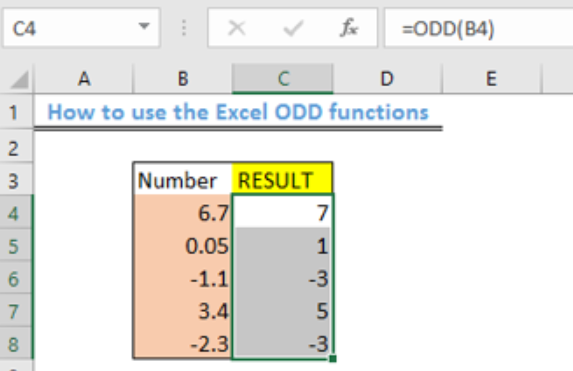Figure 1 – How to use the Excel ODD function

## General Formula

`=ODD(num)`

## Formula

`=ODD(B4)`

## Setting up the Data

We will prepare our table as shown in figure 2.

• We will place the numbers to be rounded up in Cell B4 to Cell B8
• We will label Column C as “result.”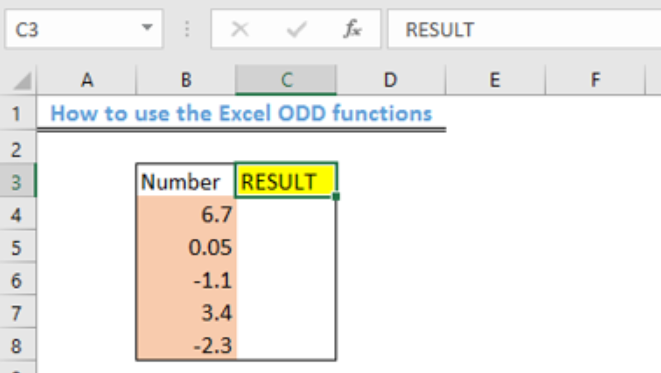Figure 2 – Setting up Data

Applying the ODD function

• We will click on Cell C4
• We will insert the formula below into Cell C4

`=ODD(B4)`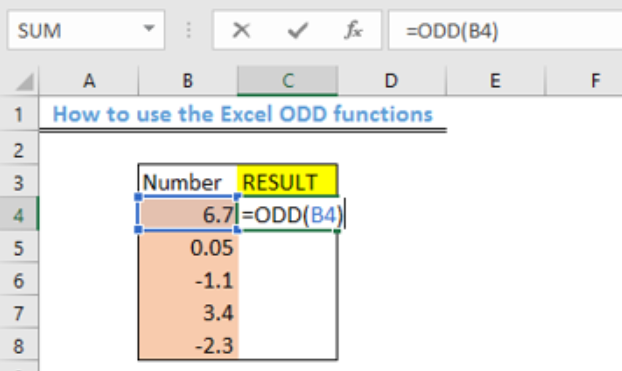Figure 3 – Applying the ODD function

• We will press the enter key to display the result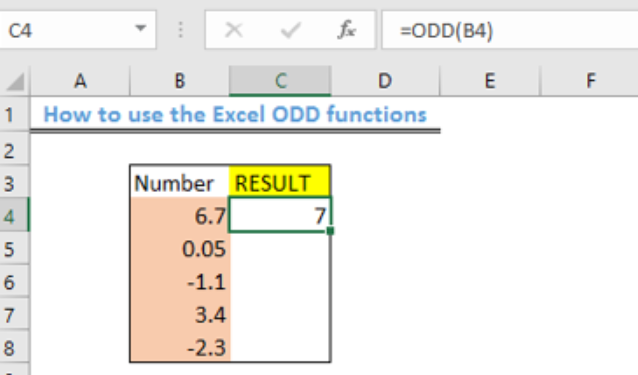Figure 4 – The Excel ODD function

• Using the fill handle, we will copy the formula to the other cells.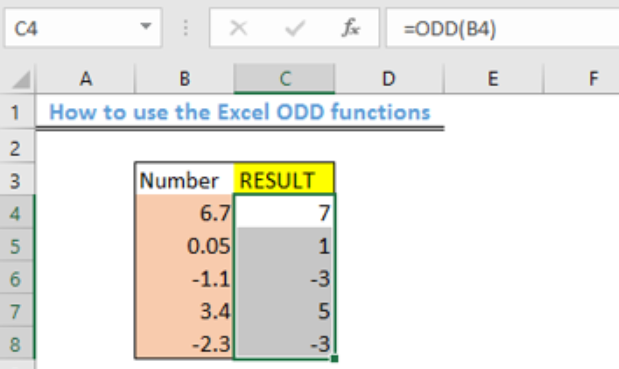Figure 5 – Result of using the ODD function

## Explanation

The ODD function always rounds up numbers to the next odd integer while skipping the even integers. When you use the ODD function with negative numbers, the value it returns with is rounded away from zero to become more negative.

## Instant Connection to an Expert through our Excelchat Service

Most of the time, the problem you will need to solve will be more complex than a simple application of a formula or function. If you want to save hours of research and frustration, try our live Excelchat service! Our Excel Experts are available 24/7 to answer any Excel question you may have. We guarantee a connection within 30 seconds and a customized solution within 20 minutes.

### Did this post not answer your question? Get a solution from connecting with the expert.Another blog reader asked this question today on Excelchat:## Subscribe to Excelchat.coAnother blog reader asked this question today on Excelchat: